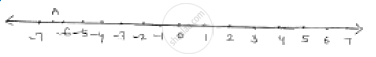# Give the Geometrical Representation of 2x + 13 = 0 as an Equation in One Variable . - Mathematics

Give the geometrical representation of 2x + 13 = 0 as an equation in one variable .

#### SolutionOne variable representation of  2x + 13 = 0

2x = -3

 x = (-13)/ 2  = -6 1/2

Points A represents   (-13) / 2

Concept: Equations of Lines Parallel to the X-axis and Y-axis
Is there an error in this question or solution?

#### APPEARS IN

RD Sharma Mathematics for Class 9
Chapter 7 Linear Equations in Two Variables
Exercise 7.4 | Q 2.1

Share